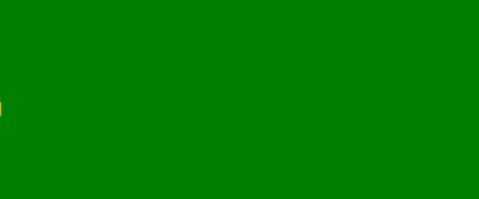# Draw moving object using Turtle in Python

#### Prerequisite: Python Turtle Basics

Turtle is an inbuilt module in python. It provides drawing using a screen (cardboard) and turtle (pen). To draw something on the screen, we need to move the turtle. To move turtle, there are some functions i.e forward(), backward(), etc.

#### 1.)Move the Object (ball)  :

Following steps are used:

• Import Turtle package.
• Set screen with dimensions and color.
• Form turtle object with color.
• Form the object (ball – made of colored circle).
• Call the function for making object again and again and clear the screen.

Below is the implementation  :

## Python3

 `# import turtle package ` `import` `turtle ` ` `  ` `  `# function for movement of an object  ` `def` `moving_object(move): ` `     `  `    ``# to fill the color in ball ` `    ``move.fillcolor(``'orange'``)  ` `     `  `    ``# start color filling ` `    ``move.begin_fill()  ` `     `  `    ``# draw circle ` `    ``move.circle(``20``)   ` `     `  `    ``# end color filling ` `    ``move.end_fill()              ` ` `  `# Driver Code ` `if` `__name__ ``=``=` `"__main__"` `: ` `     `  `    ``# create a screen object ` `    ``screen ``=` `turtle.Screen()  ` ` `  `    ``# set screen size ` `    ``screen.setup(``600``,``600``)     ` ` `  `    ``# screen background color ` `    ``screen.bgcolor(``'green'``)    ` ` `  `    ``# screen updaion  ` `    ``screen.tracer(``0``)            ` ` `  `    ``# create a turtle object object ` `    ``move ``=` `turtle.Turtle()  ` ` `  `    ``# set a turtle object color ` `    ``move.color(``'orange'``) ` ` `  `    ``# set turtle object speed ` `    ``move.speed(``0``)  ` ` `  `    ``# set turtle object width ` `    ``move.width(``2``)      ` ` `  `    ``# hide turtle object ` `    ``move.hideturtle()           ` ` `  `    ``# turtle object in air ` `    ``move.penup()                ` ` `  `    ``# set initial position ` `    ``move.goto(``-``250``, ``0``)  ` ` `  `    ``# move turtle object to surface ` `    ``move.pendown()              ` ` `  `    ``# infinite loop ` `    ``while` `True` `: ` `         `  `        ``# clear turtle work ` `        ``move.clear()   ` `         `  `        ``# call function to draw ball ` `        ``moving_object(move)    ` `         `  `        ``# update screen ` `        ``screen.update()     ` `         `  `        ``# forward motion by turtle object ` `        ``move.forward(``0.5``)       `

#### Output :

Example 2:  Move the Object (box)

Following steps are used:

• Import Turtle package.
• Set screen with dimensions and color.
• Form turtle object with color.
• Form the object (box – made of colored square).
• Call the function for making object again and again and clear the screen.

Below is the implementation:-

## Python3

 `import` `turtle ` ` `  `screen ``=` `turtle.Screen() ` `screen.setup(``500``,``500``) ` `screen.bgcolor(``'Green'``) ` ` `  `# tell screen to not  ` `# show automatically ` `screen.tracer(``0``)             ` ` `  `t ``=` `turtle.Turtle() ` `t.speed(``0``) ` `t.width(``3``) ` ` `  `# hide donatello, we ` `# only want to see the drawing ` `t.hideturtle()             ` ` `  `def` `draw_square() : ` `    ``t.fillcolor(``"Orange"``) ` `    ``t.begin_fill() ` `    ``for` `side ``in` `range``(``4``) : ` `        ``t.forward(``100``) ` `        ``t.left(``90``) ` `    ``t.end_fill() ` `t.penup() ` `t.goto(``-``350``, ``0``) ` `t.pendown() ` ` `  `while` `True` `: ` `    ``t.clear() ` `    ``draw_square() ` `     `  `    ``# only now show the screen, ` `    ``# as one of the frames ` `    ``screen.update()          ` `    ``t.forward(``0.02``) ` `     `  `# This code is contributed by pulkitagarwal03pulkit`

Output:Whether you're preparing for your first job interview or aiming to upskill in this ever-evolving tech landscape, GeeksforGeeks Courses are your key to success. We provide top-quality content at affordable prices, all geared towards accelerating your growth in a time-bound manner. Join the millions we've already empowered, and we're here to do the same for you. Don't miss out - check it out now!

Previous
Next